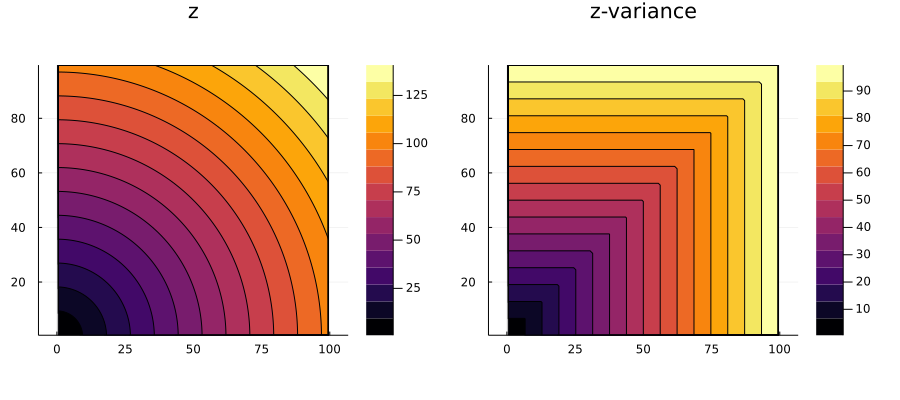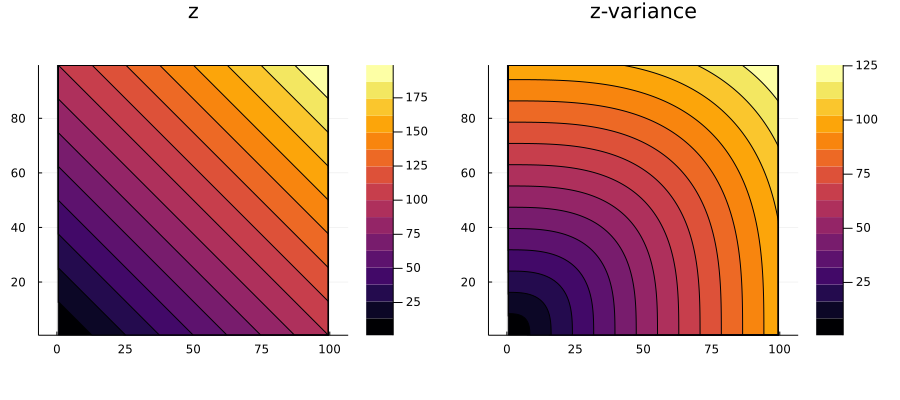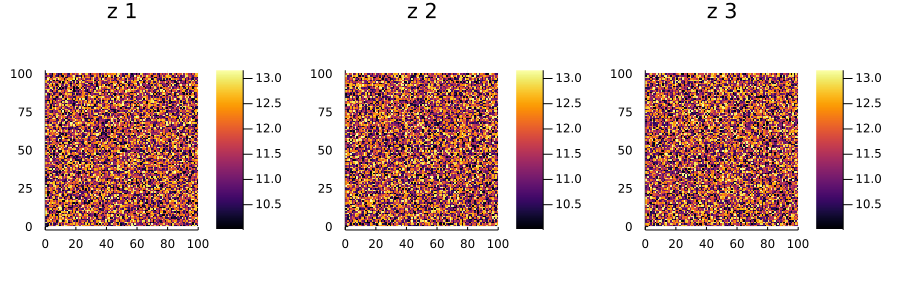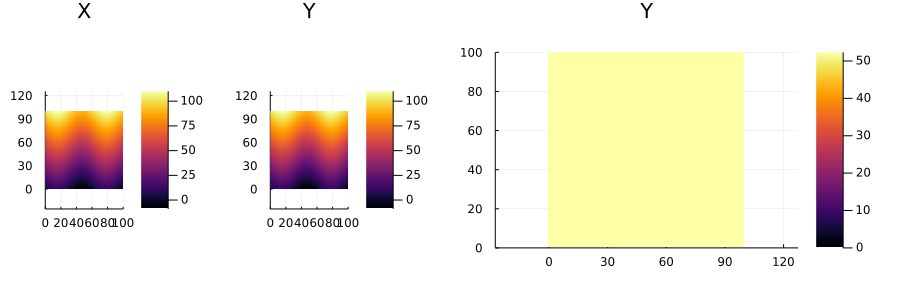# Writing solvers

After reading this guide, you should be able to write your own geostatistical solver, and enjoy a large set of features for free, including distributed parallel execution, a suite of meta algorithms, and various plot recipes. If you have any questions, please don't hesitate to ask in our gitter channel.

## Basics

Currently, there are three types of geostatistical problems defined in the framework:

EstimationProblem
SimulationProblem
LearningProblem

The task of writing a solver for a geostatistical problem consists of writing a simple function in Julia that takes the problem as input and returns the solution. In the following sections we illustrate the development process and share working examples that could be copied/pasted as starters.

### Writing estimation solvers

#### Create the package

Install the PkgTemplates.jl package and create a new project:

using PkgTemplates

generate_interactive("MySolver")

This command will create a folder named ~/user/.julia/vx.y/MySolver with all the files that are necessary to load the new package:

using MySolver

Choose a license for your solver. If you don't have major restrictions, I suggest using the MIT license. Try to choose a permissive license so that your solver can be used, and improved by private corporations.

#### Import GeoStatsBase

After the package is created, open the main source file MySolver.jl and add the following lines:

using Meshes
using GeoStatsBase
import GeoStatsBase: solve

These lines bring all the symbols defined in Meshes and GeoStatsBase into scope, and tell Julia that the method solve will be specialized for the new solver. Next, give your solver a name:

struct MyCoolSolver <: EstimationSolver
# optional parameters go here
end

and export it so that it becomes available to users of your package:

export MyCoolSolver

At this point, the MySolver.jl file should have the following content:

module MySolver

using Meshes
using GeoStatsBase
import GeoStatsBase: solve

export MyCoolSolver

struct MyCoolSolver <: EstimationSolver
# optional parameters go here
end

end # module

#### Write the algorithm

Now that your solver type is defined, write your algorithm. Write a function called solve that takes an estimation problem and your solver, and returns an estimation solution:

function solve(problem::EstimationProblem, solver::MyCoolSolver)
pdomain = domain(problem)

μs = []; σs = []
for var in name.(variables(problem))
push!(μs, var => rand(nelements(pdomain)))
push!(σs, Symbol(var,"-variance") => rand(nelements(pdomain)))
end

georef((; μs..., σs...), pdomain)
end

Paste this function somewhere in your package, and you are all set.

#### Test the solver

To test your new solver, load the GeoStats.jl package and solve a simple problem:

using GeoStats
using MySolver

sdata    = georef(CSV.File("samples.csv"), (:x,:y))
sdomain  = CartesianGrid(100, 100)
problem  = EstimationProblem(sdata, sdomain, :value)

solution = solve(problem, MyCoolSolver())

plot(solution)

### Writing simulation solvers

The process of writing a simulation solver is very similar, but there is an alternative function to solve called solvesingle that is preferred. The function solvesingle takes a simulation problem, one of the variables to be simulated, a solver, and a preprocessed input, and returns a vector with the simulation results:

function solvesingle(problem::SimulationProblem, covars::NamedTuple,
solver::MySimSolver, preproc)
# retrieve problem info
pdata = data(problem)
pdomain = domain(problem)

real4var = map(covars.names) do var
# output is a single realization for each covariable
real = Vector{Float64}(undef, nelements(pdomain))

# algorithm goes here
# ...

var => real
end

Dict(real4var)
end

This function is preferred over solve if your algorithm is the same for every single realization (the algorithm is only a function of the random seed). In this case, GeoStats.jl will provide an implementation of solve for you that calls solvesingle in parallel.

The argument preproc is ignored unless the function preprocess is also defined for the solver. The function takes a simulation problem and a solver, and returns an arbitrary object with preprocessed data:

preprocess(problem::SimulationProblem, solver::MySimSolver) = nothing

### Writing learning solvers

Similar to the other cases, writing a LearningSolver compatible with the framework consists of writing a simple Julia function that takes the LearningProblem as input along with the solver, and returns spatial data with learned variables.

## Examples

### Estimation

An estimation solver that, for each location of the domain, assigns the 2-norm of the coordinates as the mean and the ∞-norm as the variance.

using Meshes
using GeoStatsBase
using LinearAlgebra: norm

# implement method for new solver
import GeoStatsBase: solve

@estimsolver NormSolver begin
@param pmean = 2
@param pvar  = Inf
end

function solve(problem::EstimationProblem, solver::NormSolver)
pdomain = domain(problem)

# dictionary mapping variable names to types
mactypeof = Dict(name(v) => mactype(v) for v in variables(problem))

# results for each variable
μs = []; σs = []

for covars in covariables(problem, solver)
for var in covars.names
# get user parameters
varparams = covars.params[(var,)]

# get variable type
V = mactypeof[var]

# allocate memory for result
varμ = Vector{V}(undef, nelements(pdomain))
varσ = Vector{V}(undef, nelements(pdomain))

for location in traverse(pdomain, LinearPath())
x = coordinates(centroid(pdomain, location))

varμ[location] = norm(x, varparams.pmean)
varσ[location] = norm(x, varparams.pvar)
end

push!(μs, var => varμ)
push!(σs, Symbol(var,"-variance") => varσ)
end
end

georef((; μs..., σs...), pdomain)
end;
solve (generic function with 7 methods)

We can test the newly defined solver on an estimation problem:

using GeoStats
using Plots

# dummy spatial data with a single point and no value
sdata   = georef((z=[NaN],), reshape([0.,0.], 2, 1))

# estimate on a regular grid
sdomain = CartesianGrid(100, 100)

# the problem to be solved
problem = EstimationProblem(sdata, sdomain, :z)

# our new solver
solver = NormSolver()

solution = solve(problem, solver)

contourf(solution)And assess the behavior of different parameters:

solver = NormSolver(:z => (pmean=1,pvar=3))

solution = solve(problem, solver)

contourf(solution)### Simulation

A simulation solver that, for each location of the domain, assigns a random sample from a Gaussian distribution.

using Meshes
using GeoStatsBase

# implement method for new solver
import GeoStatsBase: solvesingle

@simsolver RandSolver begin
@param mean = 0
@param var  = 1
end

function solvesingle(problem::SimulationProblem, covars::NamedTuple,
solver::RandSolver, preproc)
pdomain = domain(problem)

real4var = map(covars.names) do var
# retrieve solver parameters
varparams = covars.params[(var,)]
μ, σ² = varparams.mean, varparams.var

# i.i.d. samples ~ Normal(0,1)
z = rand(nelements(pdomain))

# rescale and return
var => μ .+ sqrt(σ²) .* z
end

Dict(real4var)
end;
solvesingle (generic function with 9 methods)

We can test the newly defined solver in a simulation problem:

using GeoStats
using Plots

# simulate on a regular grid
sdomain = CartesianGrid(100, 100)

# the problem to be solved
problem = SimulationProblem(sdomain, :z => Float64, 3)

# our new solver
solver = RandSolver(:z => (mean=10.,var=10.))

solution = solve(problem, solver)

heatmap(solution)Note, however, that we did not define the preprocess function for the solver. This function can be used to avoid recalculations for each realization, and to set default parameters for variables that are not explicitly set by users in the solver constructor:

import GeoStatsBase: preprocess

function preprocess(problem::SimulationProblem, solver::RandSolver)
preproc = Dict()
for covars in covariables(problem, solver)
for varname in covars.names
varparams = covars.params[(varname,)]
preproc[varname] = (mean=varparams.mean, var=varparams.var)
end
end

preproc
end;
preprocess (generic function with 10 methods)

We can call the preprocess function on problems with multiple variables to check that the solver is producing default values for variables other than the one passed during construction:

problem = SimulationProblem(sdomain, (:z=>Float64, :w=>Float64), 3)

preprocess(problem, solver)
Dict{Any, Any} with 2 entries:
:w => (mean = 0, var = 1)
:z => (mean = 10.0, var = 10.0)

This preproc output is passed by GeoStats.jl as the last argument to the solvesingle function, which could be reimplemented as follows:

function solvesingle(problem::SimulationProblem, covars::NamedTuple,
solver::RandSolver, preproc)
pdomain = domain(problem)

real4var = map(covars.names) do var
# retrieve solver parameters
μ, σ² = preproc[var]

# i.i.d. samples ~ Normal(0,1)
z = rand(nelements(pdomain))

# rescale and return
var => μ .+ sqrt(σ²) .* z
end

Dict(real4var)
end;
solvesingle (generic function with 9 methods)

### Learning

A learning solver that copies the mean of a response variable defined in source data over to target data:

using Meshes
using GeoStatsBase

# implement method for new solver
import GeoStatsBase: solve

# new solver that copies the mean
struct MeanSolver <: LearningSolver end

function solve(problem::LearningProblem, solver::MeanSolver)
# retrieve problem info
sdata  = sourcedata(problem)
tdata  = targetdata(problem)

# mean of response over source data
μ = mean(sdata[resp])

# copy the mean over target domain
μs = fill(μ, nelements(tdata))

# new table of attributes
table = (; zip([resp], [μs])...)

# georeference new table
georef(table, domain(tdata))
end;
solve (generic function with 8 methods)

We can test the newly defined solver in a learning problem:

using GeoStats
using Plots

# create some values
v = [10sin(i/10) + j for i in 1:100, j in 1:100]

# source data has X and Y
Ωs = georef((X=v, Y=v))

# target data only has X
Ωt = georef((X=v,))

# regression from X to Y
plot(plot(Ωs), plot(μ))